Reach Us+44 1704 335730
Solving Economic Dispatch By Using Swarm Based Mean-Variance Mapping Optimization (MVMOS) | OMICS International
Global Journal of Technology and Optimization
All submissions of the EM system will be redirected to Online Manuscript Submission System. Authors are requested to submit articles directly to Online Manuscript Submission System of respective journal.

# Solving Economic Dispatch By Using Swarm Based Mean-Variance Mapping Optimization (MVMOS)

Truong Khoa H1*, Vasant P1, Balbir Singh1 and Vo Dieu N2

1Department of Fundamental and Applied Sciences, Universiti Teknologi Petronas, Malaysia

2Department of Power Systems, HCMC University of Technology, Vietnam

Corresponding Author:
Dr. Truong H Khoa
Department of Fundamental and
Applied Sciences, Universiti Teknologi
Petronas, Malaysia
Tel:
+60 5-368 8000
E-mail: [email protected]

Received Date: April 11, 2015 Accepted Date: June 29, 2015 Published Date: July 06, 2015

Citation: Khoa TH, Vasant P, Singh B, Dieu VN (2015) Solving Economic Dispatch By Using Swarm Based Mean-Variance Mapping Optimization (MVMOS). Global J Technol Optim 6:184. doi:10.4172/2229-8711.1000184

Copyright: © 2015 Khoa TH, et al. This is an open-access article distributed under the terms of the Creative Commons Attribution License, which permits unrestricted use, distribution, and reproduction in any medium, provided the original author and source are credited.

Visit for more related articles at Global Journal of Technology and Optimization

#### Abstract

This paper proposes a novel optimization which named as Swarm based Mean-variance mapping optimization (MVMOS) for solving the economic dispatch. The proposed optimization algorithm is the extension of the original single particle mean-variance mapping optimization (MVMO). The novel feature is the special mapping function applied for the mutation base on the mean and variance of n-best population.The MVMOS outperforms the classical MVMO in global search ability due to the improvement of the mapping. The proposed MVMOS is investigated on four test power systems, including 3-, 13- , 20- thermal generating units and large-scale system 140 units with quadratic cost function and the obtained results are compared with many other known methods in the literature. Test results show that the proposed method can efficiently implement for solving economic dispatch.

#### Keywords

Economic dispatch; Quadraticfuel cost function; MVMO; MVMOS

#### Nomenclature

N                     Total number of generator

FT                    Total operationcost

Fi                      Fuel cost function of generator i

ai, bi, ci             Fuel cost coefficients of generator i

Bij,B0i,B00        B-matrix coefficients for transmission power loss

Pi                     Power output of generatori

Pi,max               Maximum power output of generator i

Pi,min                Minimum power output of generator i

PL                    Total transmission loss

itermax              Maximum number of iterations

n_var               Numberof variable (generators)

n_par               Number of particles

mode               Variable selection strategy for offspring creation

archive zize       n-best individuals to be stored in the table

di                     Initial smoothing factor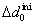Initial smoothing factor increment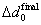Final smoothing factor increment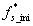Initial shape scaling factor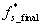Final shape scaling factor

Dmin                 Minimum distance threshold to the global best solution

n_randomly      Initial number of variables selected for mutation

indep.runs         m steps independently to collect a set of reliable individual solutions

#### Introduction

The Economic Dispatch (ED) is an essential optimization task in the power generation system and its objective is to determine the economical real power output of the thermal generating units to supply required power load demand at the minimum fuel cost while satisfying all units and system constrains [1,2]. Since the concept of economic dispatch (ED) started in the 1950’s, there are a lot of various methods have been employed for solving ED problems, but in short there are three main categories: Methods based on mathematical programming (Classical calculus-based techniques), methods based on artificial intelligence and hybrid methods.

For mathematical convenience, the objective cost function of ED problem is the quadratic function approximations , was solved by methods based on mathematical programming such as lambda iteration method, Newton’s method, gradient search, dynamic programming , linear programming , non-linear programming  and quadratic programming . These methods are conventional techniques that were early employed. Over the past years, more advanced methods based on artificial intelligence have been developed and implemented outstandingly to ED problem such as Hopfield Neural Network (HNN) [7,8], Evolutionary Programming (EP) , Differential Evolution (DE) , Genetic Algorithm (GA) , Ant Colony Optimization (ACO) , Particle Swarm Optimization (PSO) [13,14] Bacterial Foraging (BF) , and Artificial Bee Colony (ABC) algorithm . These methods do not always guarantee to find the global optimal solution in finite computational time but their ability often find near global optimal solution for optimization problems. Besides the single mentioned methods, hybrid methods have been also developed for solving the ED problems such as hybridization of evolutionary programming with Sequential Quadratic Programming (EP-SQP) , combining of chaotic differential evolution and quadratic programming (DEC-SQP)  hybrid technique integrating the uniform design with the genetic algorithm (UHGA) , self-tuning hybrid differential evolution (selftuning HDE) , and fuzzy adaptive particle swarm optimization algorithm with Nelder–Mead simplex search (FAPSO-NM) . These hybrid methods become powerful search methods for obtaining higher solution quality due to using the advantages of each element method to improve their search ability for the complex problems. Nevertheless, they may be slower and more complicated than the element methods because of combination of several operations.

The above artificial intelligence methods are population based meta-heuristic which can deal with multiform optimization problems . Recently, Prof. István Erlich has been conceived and developed a novel optimization technique which is named Mean-variance mapping optimization (MVMO) . This algorithm is so-called “populationbased stochastic optimization techniques”. MVMO has the capability to find the optimum solution quickly with minimum risk of premature convergence.

The extensions of MVMO, which named Swarm based Meanvariance mapping optimization (MVMOS) , has been developed to become more effective. In this paper, MVMOS is proposed for solving the economic dispatch problem with quadratic cost function.

Section II presents the formulation of the ED. The review of MVMO, extension of MVMO-MVMOS and implementation of the proposed MVMOS to ED problem are addressed in Section III. The numerical results are showcased in Section IV. The discussion is followed in Section V. After all, the conclusion are given.

#### Problem Formulation

The power system consists of N thermal generating units. Each unit has a fuel cost function, shown as Fi , togenerates a power out Pi. The total fuel costof the system,FT,is sum of fuel cost of each unit.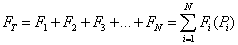(1)

The optimization problem of the ED is to minimize the total fuel cost FT which be written as: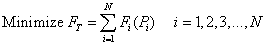(2)

Generally, the fuel cost curve of a thermal generating unit is presented as quadratic function as: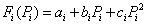(3)

The constraints of the ED problem must be satisfied during the optimization process are peresented as follows:

Real power balance equation

The total active power output of generating units must be equal to total active power load demand plus power loss: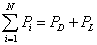(4)

The power loss PLis calculated by the below formulation :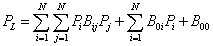(5)

Generator capacity limits

The active power output of generating units must be within the allowed limits: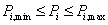(6)

#### MVMOS for Eonomic Dispatch

Review of MVMO

Mean-variance mapping optimization (MVMO) is a novel optimization algorithm falls into the category of the so-called “population-based stochastic optimization technique”. The similarities between MVMO and the other known stochastic algorithms are three evolutionary operators: selection, mutation and crossover. However, the major differences between MVMO and other existing techniques are as follows:

- The key feature of MVMO is a special mapping function which applied for mutating the offspring based on mean-variance of the solutions stored in the archive.

The mean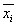and variance vi are calculated as follows: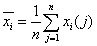(7)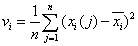(8)

where, j= 1,2,..., n (n is population size).

The mapping function is depicted in Figure 1. The transformation mapping function, h, is calculated by the mean x and shape variables si1 and si2 as follows: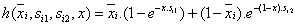(9)

where,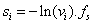(10)

The scaling factor fs is a MVMO parameter which allows for controlling the search process during iteration. si is the shape variable.

• All variables are initialized within the limit range [0,1]. The output of mapping function is always inside [0,1]. However, the function evaluation is carried out always in the original scales.

• MVMO is a single-agent search algorithm because it uses a single parent-offspring in each iteration. Therefore, the number of fitness evaluations is identical to the number of iterations.

Interested readers can find the basics of algorithm and reference values for the algorithm’s settings in [23,24].

Extension of MVMO-MVMOS

Recently,theswarm version of MVMO has been developed. This version is abbreviated as MVMOS. The new approach extends the ability of global searching of the classical MVMO by starting the search with a set of particles.

Modified version of MVMO: The MVMO-algorithm extends two important parameters. These two parameters are used for calculation and assignment of si1 and si2 as follows:

Variable FS factor: In (10), the factor fs allows the modification of the shape factor calculated from the variance.

The extension of fS factor is for the need of exploring the search space at the beginning more globally whereas, at the end of the iterations, the focus should be on the exploitation. It is determined by: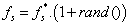(11)

Where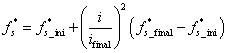(12)

r and () is a random number with the bounds [0, 1].

In (12) , the variable i represents the iterationnumber.

For the more accuracy of the optimization ,the initial and final values of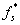it is recommended that<1 and>1 The suggested range of initial values ofis from 0.9 to 1.0 and forfinal values ofis from 1.0 to 3.0 .

When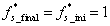, which means that the option for controlling the fs factor is not used.

Variable increment Δd : The MVMOS algorithm uses the factor Δd as presented below:

si1=si2=si

if si> 0 then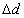= (1+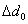)+ 2 .+(rand() – 0.5)

if si>di

di=di .Δd

else

di=di /Δd

end if

if rand() ≥ 0.5 then

si1=si ; si2=di

else

si1=si ; si2=di

end if

end if

The extension of variable increment Δd is used for the asymmetric characteristic of the mapping function.

At the start ofthe algorithm, the initial values of di(typically between 1-5) are set for all variables. At every iteration, if si>di , di will be multiplied by Δd leads to increased di. In case si<di, the current diis divided byΔd which is always greater than 1.0 and thus resulting in reduced value of di. Therefore, di will always oscillate around the current shape factor si. Furthermore, Δd is varied randomly around the value(1 + 0 Δd ) with the amplitude of 0 Δd adjusted in accordance to (14), where 0 Δd can be allowed to decrease from 0.4 to 0.01.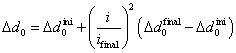(14)

Swarm variant of MVMO: Compared with classical MVMO, the swarm variant explores the solution space more aggressively. The search process is started with a set ofparticles, each having its own memory defined in terms of the corresponding archive and mapping function. Initially, each particle performs m steps independently to collect a set of reliable individual solutions. Then, the particles start to communicate and to exchange information.

The scheme of MVMOS is depicted in Figure 2.

i and k donate the function evaluation and particle counters, respectively. Whereas m and np stand for maximum number of independent runs and total number of particles, respectively.

It is not worth it to follow particles which are very close to each other since this would entail redundancy. To avoid closeness between particles (i.e. redundancy), the normalized distance of each particle’s local best solution xlbest,i to the global best xgbest is calculated by: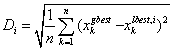(15)

where, n denotes the number of optimization variables.

The i-th particle is discarded from the optimization process if the distance Di is less than a certain user defined threshold Dmin.

A zero threshold means that all particles are considered throughout the whole process whereas a unit threshold will result in the dropping of all particles except the global best. In this case after (m*np+ np) fitness evaluations only one particle, the gbest, remains. Intermediate threshold values entail better adaptation to any optimization problem.

After independent evaluation, and if the particle is further considered, the global best solution guides the search by assigning xgbest, instead of xlbest,i, as parent for every particle’s offspring. The remaining steps are identical with those of the classical MVMO: A subset of dimensions in the parent vector is directly inherited whereas the remaining dimensions are selected and mutated, based on local statistics (mean and variance) of the particle, via mapping function.

Implemention of MVMOS to ED

Handing of constraints: To guarantee that the equality constraint (4) is always satisfied, a slack generating unit is randomlyselected from N generating units and therefore its power output will be dependent on the power outputs of remaining N-1 generating units in the system. The method for calculation of power output for the slack unit is given in . The power output of the slack unit is as follows: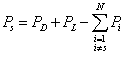(16)

where,s is a random unit selected from N units

The power transmission loss in (5) is rewritten by considering PS as an unknown variable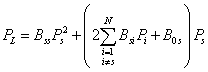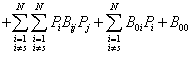(17)

Substituting (17) into (13), a quadratic equation is abtained as follows: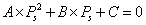(18)

where A, B and C are given by: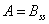(19)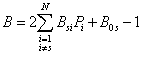(20)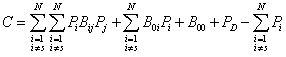(21)

The power output of the slack generator is the posstive root of (18) between the two ones abtained as follows: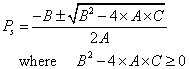(22)

Based on the slack variable method, the fitness function for the proposed MVMOS will include the objective function (2) and penalty terms for the slack unit if inequality (6) is violated. The fitness function is as follows: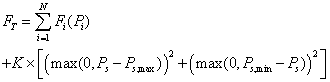(22)

Implemention of MVMOS to ED: The steps of procedure of MVMOS for the ED problem are described as follows:

Step 1: Setting the parameters for MVMOS including itermax, n_var, n_par, mode, di,, archive zize,,n_randomly, n_randomly_min, indep.runs(m), Dmin

Set i=1, i donates the function evaluation

Step 2: Normalize initial variables to the range [0,1] (i.e. swarm of particles).

x_normalized= rand(n_par,n_var)

Step 3: Set k=1, kdonate particle counters.

Step 4: Using de-normalized variables to evaluate fitness function, store fbestand xbestin archive

Step 5: Increase i =i+1. If i<m ( independent steps), go to Step 5. Otherwise, go to Step 6.

Step 6: Check the particles for the global best, collect a set of reliable individual solutions. The i-th particle is discarded from the optimization process if the distance Di is less than a certain user defined threshold Dmin.

Step 7: Create offspring generation through three evolutionary operators: selection, mutation and crossover.

Step 8: if k<np ,increasek=k+1 and go to step 4. Otherwise, go to step 9.

Step 9: Check termination criteria. If stoping criteria is satisfied, stop. Otherwise, go to step 3.

De-nomalization of optimization variables: The output of mapping function is always inside [0,1]. However, the function evaluation is carried out always in the original scales. De-nomalization of optimization variables is carried by using (24):

Pi=Pi,min + Scaling.x_normalized(ι,:)

where,

Scaling=Pi,max -Pi,min

Termination criteria:The algorithm of the proposed MVMOS is terminated when the maximum number of iterations itermax is reached.

#### Numerical Analysis

The proposed MVMOS has been applied to the ED problem with the quadratic cost function.Four test cases including 3, 13, 20 thermal generating units and large-scale systemwith 140 units are carried out. For each case, the algorithm of MVMOS is run 50 independent trialsona Intel Core i5-3470 CPU 3.2 GHz PC, Ram 4GB. The implematation of the proposed MVMOS was done in Matlab R2013a platform.

Selection of parameters

The parameters of MVMOSinclude itermax, n_var, n_par, mode, di,,,archive zize,,n_randomly, indep.runs(m), Dmin. Since different parameters of the proposed method effect on the performance of MVMOS. Hence, it is important to determine an optimal set of parameters of the proposed methods for ED problem. For each problem, selection of parameters is carried out by varying only one parameter at a time and keeping the other. The parameter is first fixed at the low value and then increased. Multiple runs are carried out to choose the suitable set of parameters. The typical parameters are selected as follows:

• itermax : maximum number of iterations depend on the dimension of problems. The maximum number of iterations is selected in the range from 1000 to 50000 iterations for case 1, case 2 and case 3, and 80000 for case 4.

• n_var: number of variable (generators), dimension of problems. n_var is set to 3,13, 20, 140 for case 1, case 2, case 3and case 4, respectively.

• n_par: number of particles is varied from 5, 10, 20, 30, 40 and 50, respectively. By experiments, the good solution is obtained when number of particles isset to 5. Hence, number of particles is set for all cases.

• mode: There are four variable selection strategy for offspring creation . Afer all simulations, stragy 3 (mode=4) is suporior to the other stragy.,The range ofin (14) is [0.01-0.4]. By experiments,andis set to 0.4 and 0.02, respectively for all cases.,Therange of values ofis from 0.9 to 1.0 and forvalues ofis from 1.0 to 3.0 . For all cases,is set to 0.95 in the range [0.9, 1.0] andis set to 3 in the range 3 in the range [1.0, 3.0].

indep.runs(m) : The maximum number of independent runs can be selected in the range from 100 to 800.

D min is set to 0 for all cases.

Numerical results

Case 1: 3 unit -system: The test system consists of 3 generating units without transmission loss. Here, the system load demand is 450MW and 850MW, respectively. The data of the system is taken from . The power transmission loss is neglected in this case. The obtained results by the MVMOS corresponding to the two load demand are given in Table 1.

Unit Power outputs Pi (MW)
PD= 450MW         PD= 850MW
1 205.3077 393.1698
2 183.3457 334.6038
3 61.3466 122.2264
Total power(MW) 450 850
Min Cost (\$/h) 4652.4735 8194.3561
Average CPU time (s) 0.87 0.88

Table 1: Power output of 3-unit system for load demand of 450 MW and 850 MW by MVMOS.

The parameters for MVMOS are set as follows: itermax=1000, n_var(generators)=3, np=5, archive size=4, indep.runs (m)=100, n_randomly=2, n_randomly_min=2,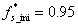,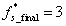,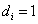,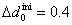,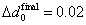,Dmin=0

The total cost comparison between MVMOS and the other methods are presented in Table 2. In case ofthe 450MW load demand, the results and computational time of MVMOS are less than PSO and ABC. In case ofthe 850MW load demand, the results ofMVMOS is less than IEP, HS, GA, BGA, and the same as NM, PSO. The proposed MVMOS is faster than HS, GA and BGA. There is no computer processor reported for PSO, ABC, HS, GA and BGA and no computational time for the other methods. Table 1 shows that the power output obtained by the MVMOS is always satisfy the constraints.

Method 450 (MW) 850 (MW)
Cost (\$/h) CPU (s) Cost (\$/h) CPU (s)
PSO 4653 7.69 - -
ABC 4653 3.91 - -
NM - - 8194.3561 -
IEP - - 8194.3561 -
PSO - - 8194.3561 -
HS - - 8194.5 27.62
GA - - 8194.3591 10.94
BGA - - 8194.357 3.66
MVMOS 4652.4735 0.87 8194.3561 0.88

Table 2: Comparison of results and CPU time by MVMO and other techniques for 3-unit system.

Case 2: 13 unit - system: The data of 13 generating unit test system is from . In this case, the power transmission loss is neglected. The obtained results by the MVMOS corresponding to the twoload demand of 1800MW and 2520MW are shown in Table 3.

Unit Power outputs Pi (MW)
PD= 1800MW    PD= 2520MW
1 506.9117 679.9970
2 253.4558 359.9957
3 253.4560 360.0000
4 99.3627 155.1418
5 99.3627 155.1929
6 99.3627 155.0396
7 99.3628 156.0892
8 99.3628 154.4661
9 99.3627 154.0705
10 40.0000 40.0015
11 40.0000 40.0038
12 55.0000 55.0022
13 55.0000 55.0000
Total power (MW) 1800.0000 2520.0000
Min Cost (\$/h) 17932.4741 24050.1408
Average CPU time (s) 2.97 16.29

Table 3: Power output of each generating unit in 13-unit system for load demand of 1800 MW and 2520 MW by MVMOS.

For the load demand of 1800MW, the parameters for MVMOS are set as follows: itermax=5000, n_var(generators)=13, npnp=5, archive size=4, indep.runs (m)=300, n_randomly=5, n_randomly_min=4,,,,,,Dmin=0

For the load demand of 2520MW, the parameters for MVMOS are set as follows: itermax=30000, n_var(generators)=13, np=5, archive size=5, indep.runs (m)=300, n_randomly=8, n_randomly_min=4,,,,,Dmin=0

The results of MVMOS for 1800 MW and 2520 MW load demands are compared to the other methods as presented in Table 4. In case of the 1800 MW load demand, the total cost obtained by MVMOS is less than HS, GA and BGA. The computational time of MVMOS is less than HS, GA and slower than BGA. There is no computer processor reported for HS, GA and BGA. In case of the 2520 MW load demand, the total cost obtained by MVMOS is less than-Iteration, GA and SQP and same result as ALHN. The computational time of MVMOS is slower than these methods. The computational times for-Iteration, GA, SQP and ALHN methods were from a Petium M 1.5 GHz PC. Table 3 shows that the power output obtained by the MVMOS is always satisfy the constraints

Method 1800 (MW) 2520 (MW)
Cost (\$/h) CPU(s) Cost (\$/h) CPU(s)
HS 18274.0065 16.135 - -
GA 18194.9507 5.8 - -
BGA 17971.5503 1.98 - -
λ-Iteration - - 24058.27 0.85
GA - - 25087.45 1.76
SQP - - 24058.29 4.57
ALHN - - 24050.14 0.044
MVMOS 17932.4741 2.97 24050.14 16.29

Table 4: Comparison of results and CPU time by MVMO and other techniques for 13-unit system.

Case 3: 20 unit-system: The test system includes 20 generators with the system load demand of 2500MW. The data of this system is from . The power transmission loss is ignored in this case. The obtained results by the MVMOS is shown in Table 5.

Unit Power outputs Pi (MW) Unit Power outputs Pi (MW)
1 600.0002 11 286.9466
2 131.1723 12 432.7209
3 50.0000 13 124.1584
4 50.0000 14 73.3046
5 92.9882 15 94.8873
6 20.0000 16 36.2083
7 125.0000 17 30.0000
8 50.0000 18 37.5181
9 111.7012 19 77.8376
10 45.5563 20 30.0000
Total power (MW) 2500.0000
Min Cost (\$/h) 60152.5509
Average CPU time (s) 31.45

Table 5: Power output of 20-unit system for load demand of 2500MW by MVMOS

The MVMOS is run 50 independent trials. The parameters for MVMOS are set as follows: itermax=70000, n_var(generators)=5, np=5, archive size=4, indep. runs(m)=400, n_randomly=7, n_randomly_ min=6,,,,,,Dmin=0

Table 6 shows the comparison of results obtained and computational time by MVMOS and the other methods. In this case, the results of MVMOS is less-Iteration, GA and SQP and the same as ALHN. The computation time of MVMOS is less than GA and slower than other methods. The computational times for-Iteration, GA, SQP and ALHN methods were from a Pentium M 1.5 GHz PC. Table 5 shows that the power output obtained by the MVMOS is always satisfy the constraints. Although the parameters for two load demands is different, the MVMOS guarantees the convergence to the global solution for the 13-unit test system.

Method Total CostPD= 2500MW CPU(s)
λ-Iteration  60245.67 0.32
GA 61107 61.17
SQP 60693.14 1.28
ALHN 60152.55 0.076
MVMOS 60152.55 31.45

Table 6: Comparison of results and CPU time by MVMO and other techniques for 20-unit system.

Case 4: large-scale system 140 unit: The Korean power system consists of 140 thermal generating units is the test system for this case. Here, the system load demand is 49342 MW. The data of the system is given in . The power transmission loss is also ignored in this case. The parameters for MVMOS are set as follows: itermax=80000, n_var(generators)=140, np=5, archive size=4, indep. runs (m)=800, n_randomly=20, n_randomly_min=10,,,,,,Dmin=0

The obtained results and computational time by the MVMOS are given in Table 7. As seen in Table 7, the power output obtained by the MVMOS is always satisfy the constraints.

Unit Pi (MW) Unit Pi (MW) Unit Pi (MW)
1 113.6343 47 239.5319 94 984.0000
2 189.0000 48 250.0000 95 978.0000
3 190.0000 49 250.0000 96 682.0000
4 190.0000 50 250.0000 98 720.0000
5 170.4101 51 165.0000 99 718.0000
6 190.0000 52 165.0000 100 720.0000
7 490.0000 53 165.0000 101 964.0000
8 490.0000 54 165.0000 102 958.0000
9 496.0000 55 180.0000 103 1007.0000
10 496.0000 56 180.0000 104 1006.0000
12 496.0000 57 103.0000 105 1013.0000
13 496.0000 58 198.0000 106 1020.0000
14 506.0000 59 312.0000 107 954.0000
15 509.0000 60 279.9162 108 952.0000
16 506.0000 61 163.0000 109 1006.0000
17 505.0000 62 95.0000 110 1013.0000
18 506.0000 63 160.0000 111 1021.0000
19 506.0000 64 160.0000 112 1015.0000
20 505.0000 65 490.0000 113 94.0000
21 505.0000 66 196.0000 114 94.0000
22 505.0000 67 490.0000 115 94.0000
23 505.0000 68 490.0000 116 244.0000
24 505.0000 69 130.0000 117 244.0000
25 505.0000 70 280.6907 118 244.0000
26 537.0000 71 137.0000 119 95.0000
27 537.0000 72 334.031 120 95.0000
28 549.0000 73 195.0000 121 116.0000
29 549.0000 74 175.0000 122 2.0000
30 501.0000 75 175.0000 123 4.0000
31 501.0000 76 175.0000 124 15.0000
32 506.0000 77 175.0000 125 9.0000
33 506.0000 78 330.0000 126 12.0000
34 506.0000 79 531.0000 127 10.0000
35 506.0000 80 531.0000 128 112.0000
36 500.0000 81 350.0494 129 4.0000
37 500.0000 82 56.0000 130 5.0000
38 241.0000 83 115.0000 131 5.0000
39 241.0000 84 115.0000 132 50.0000
40 774.0000 85 115.0000 133 5.0000

Table 7: Power output of 140-unit system for load demand of 49342MW by MVMOS.

Table 8 shows the comparison of results and computational time obtained by MVMOS and the other methods. In this case, the results of MVMOS is less than CTPSO, CSPSO, COPSO, CCPSO and KVMO. The computation time of MVMOS is slower than these methods. The computational times for CTPSO, CSPSO, COPSO and CCPSO were from Pentium IV 2.0-GHz computer.

Method Best Total Cost CPU
PD= 49342MW (s)
CTPSO  1655685 50.1
CSPSO 1655685 9.6
COPSO 1655685 76.9
CCPSO 1655685 42.9
KMVO  1577607 -
MVMOS 1557461 105.39

Table 8: Comparison of results and CPU time by MVMOS and other techniques for 140-unit system.

Robustness analysis

The convergence of heuristic methods may not obtain exactly same solution because these methods initialize variables randomly at each run. Hence, their performances could not be judged by the results of a single run. Many trials should be carry out to reach a impartial conclusion about the performance of the algorithm. Therefore, in this study, 50 independent trials were carried out. The mean cost, max cost, average cost and standard deviation obtained by the proposed method to evaluate the robustness characteristic of the proposed method for ED problem. The robustness analysis of four cases test are presented in (Tables 9 and 10).

Case 1: 3 unit Case2: 13 unit
450(MW) 850(MW) 1800(MW) 2520(MW)
Min Cost (\$/h) 4652.4735 8194.3561 117932.4741 24050.1408
Average Cost (\$/h) 4652.4735 8194.3561 17932.4741 24050.277
Max Cost (\$/h) 4652.4735 8194.3561 17932.4741 24050.189
Standard deviation (\$/h) 0 0 0 0.0309

Table 9: Robustness analysis of the proposed MVMOS by 50 independent trials for Case 1 and Case 2.

Case 3: 20 unit Case 4 : 140 unit
2500 (MW) 49342(MW)
Min Cost (\$/h) 60152.5509 1557461.803
Average Cost (\$/h) 60153.2215 1557481.743
Max Cost (\$/h) 60152.7388 1557481.743
Standard deviation(\$/h) 0.1469 3.0107

Table 10: Robustness analysis of the proposed MVMOS by 50 independent trials for Case 3 and Case 4.

Tables 9 and 10 clearly show that the performance the proposed MVMOS is very robust.

#### Results and Discussions

A solution of optimization techniques needs concern with two elements:

• Quality of solution: the quality of solution need robustness, near or better the global solutions of the other techniques.

In addition, it takes note the large-scale system problem. The computation time of technique on the large-scale system may be take more time but the quality of solution need optimum.

Based on the numerical results and robustness analysis of the proposed method, it indicates that the MVMOS obtained the global solution with high probability, especially for large-scale system due to the global search capability is enhanced. Besides its ability, the proposed MVMOS is also easy to be implemented for ED problem. Unlike other swarm-based optimization techniques, MVMOS does not strictly require many particles to progess. In this study, number of particles is set to 5 for all cases. The MVMOS showed the good performance. However, the computation time is relatively high for large-scale system. Similar to original MVMO, the number of iterations in MVMOS is equivalent to the number of offspring fitness evaluations which is in practical applications usually comsume more time than the optimization algorithm itself.

In future, the MVMOS is proposed for solving the non-convex ED problems with complicated objective function.

#### Conclusions

In this paper the proposed MVMOS has been tested for the ED problem with quadratic cost function efficiently and effectively.The numerical results show that the MVMOS exhibits a robust performance and also provides the good solutions for all test systems, expecially for lagre-scale system. The proposed method has merits as follows: easy implementation, good solutions, robustness of algorithm; applicable to large-scale system. Therefore, the proposed MVMOS could be favorable for solving other ED problems.

#### Acknowledgment

This research work is financially supported by Graduate Assistant Scheme (GAS) of Universiti Teknologi PETRONAS and with the help of Department of Fundamental and Applied Sciences, Faculty of Science and Information Technology, UTP.

#### References

Select your language of interest to view the total content in your interested language

### Article Usage

• Total views: 13256
• [From(publication date):
December-2015 - Nov 12, 2019]
• Breakdown by view type
• HTML page views : 9250Can't read the image? click here to refresh